Rotated 1D Problem

 As shown in the picture, a 1D problem defined along the direction which has an non-zero angle with respect with respect to x-direction. Computation is conducted in x-y coordinate (in region OABC ).The physical variables in x-y coordinate are obtained from those in the new coordinate, and vice versa. This is often employed to test numerical methods for keeping constraint of for 2D problems.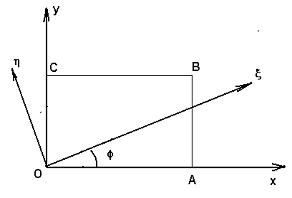Smooth Alfven Wave problem

 Initial condition: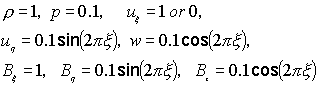r=5/3       ux=1 for standing wave problem       ux=0 for moving wave problem Computational Domain: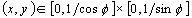Rotated Angle: 60° Computational time: t=5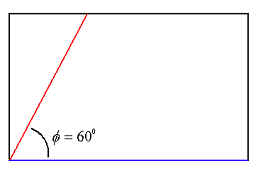Standing wave problem Moving wave problem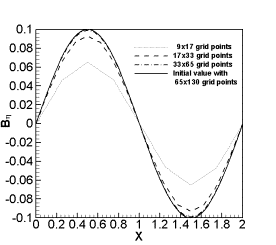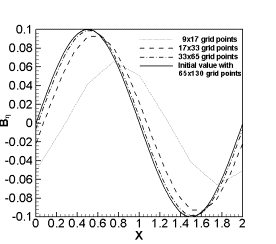Profile of component Bh is plot along line y = 0 (blue line in the plot of computational domain)

MHD Shock tub problem

 Rotated angle:  f=45° Computational domain: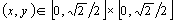Computational time: t=0.1 Mesh: 399x799 grid points  Initial condition: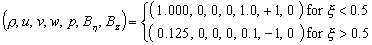with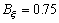and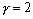.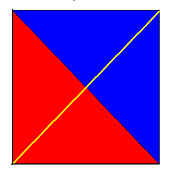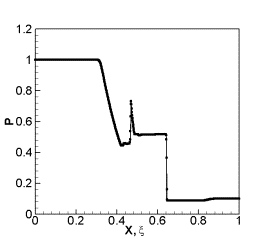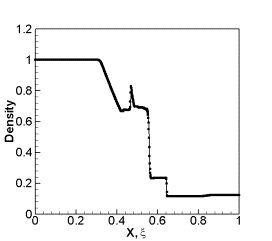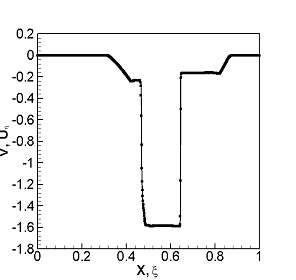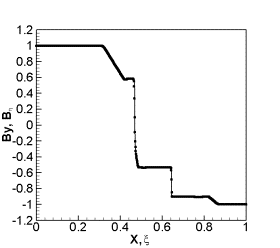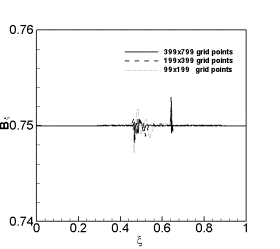In above figures, dots stand for 2D results and lines for 1D results.

Movie: evolution of component Bx  along x direction (yellow line).